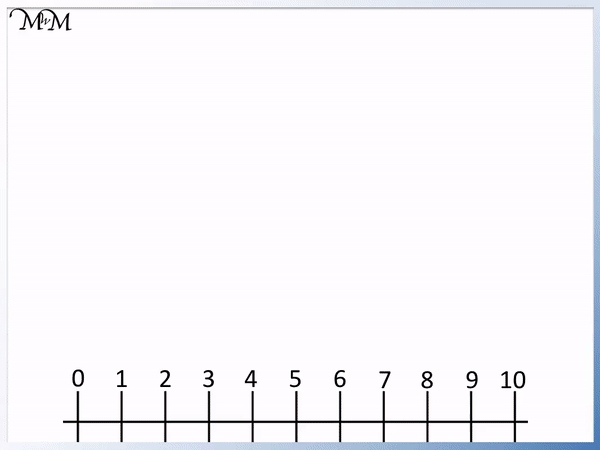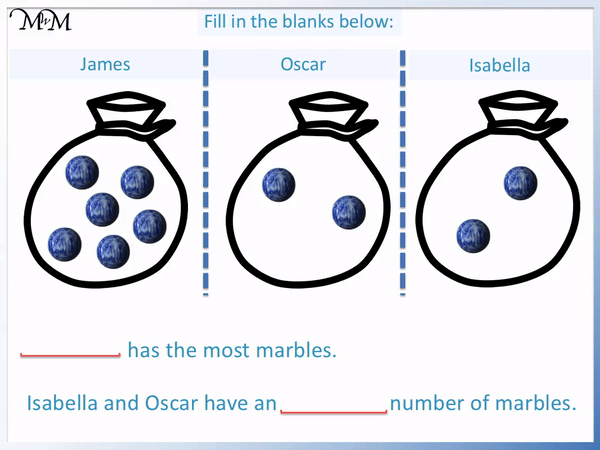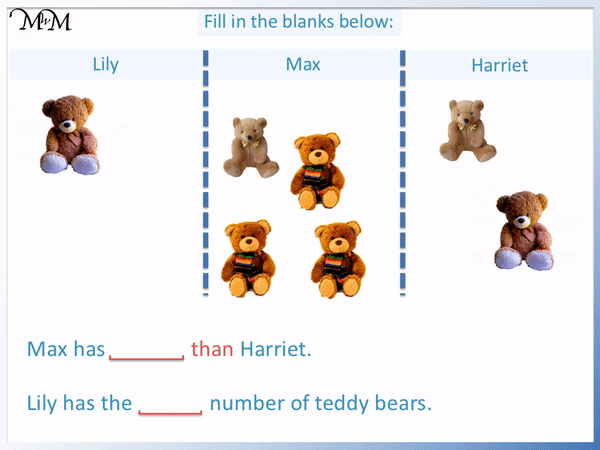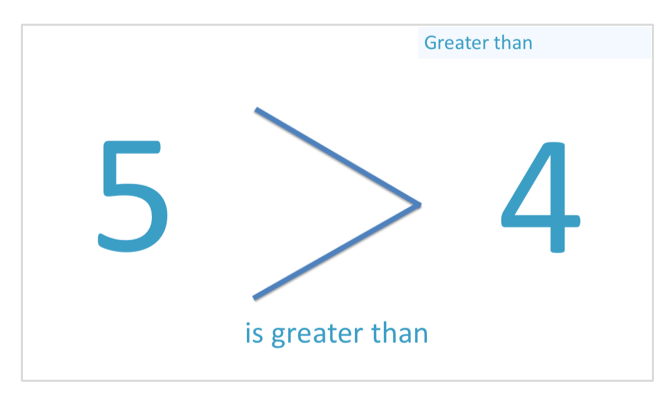# Teaching More or Less Than for Comparing Quantities

Teaching More or Less Than for Comparing Quantities• We are comparing the number of teddy bears to write who has more or less:
• When teaching more or less, it is first important to have a strong understanding of the concept of number order and counting.
• As you count each item, we can move along one place on a number line.
• We can teach that the biggest number is the number that is furthest along the number line.
• 5 is more than 3 and so I have more than my friend.
• We can also compare quantities by pairing them up as shown.
• This helps us to see that I have 2 more than my friend.

We can count on a number line to compare quantities using numbers.

We can pair objects by lining them up to see who has the most.• We are comparing the marbles below by counting them and writing down the number of each.
• James has 6, Oscar has 2 and Isabella has 2.
• The biggest number is 6 and so James has the most.
• Oscar and Isabella both have 2 marbles, the same number.
• The word equal can be used to mean the same number as.# How to Teach Comparing Quantities using More than and Less than

This is a lesson introducing the comparison of quantities to children by using the words more than, less than and equal to.

When teaching the comparison of quantities, it is first important for a child to have a good understanding of number order and this can be taught by using a number line.

Once the order of numbers can be visualised in a sequence, then we can move on to practise counting objects.

Below is our first example.We will count the number of my marbles and count the number of my friend’s marbles.

I have 1 marble.

My friend has 4 marbles.

We know that 4 is a bigger number than 1 because we know the sequence of numbers and know that 4 comes after 1.

However it may help to reinforce the comparative size of each number using a number line.

More means bigger.

Since the number of marbles that my friend has is a bigger number than my number of marbles, we say that:

My friend has more than me.

The opposite of more than is less than

I have less than my friend.

Here is another example:We will compare the amounts of marbles by counting.

I have 3 marbles and my friend has 2 marbles.

3 is after 2 and so it is a bigger number.

We can see that 3 is further along the number line than 2 is.

I have a bigger number of marbles than my friend.

I have more marbles than my friend.

My friend has less marbles than my friend.

Here is another example of teaching more than or less than.

One way to help teach more than or less than is to line the items up alongside each other.Counting the number of my bears, I have 5.

My friend has 3 bears.

We can teach the concept of more than by pairing off one of each object at a time.

We can see that having paired off all of the three of my friend’s bears, I still have some left.

I have more than my friend.

We can see that I have 2 more than my friend.

In this new example below, my friend is given some more teddy bears.

Who has the most now?I still have the same number of teddies.

I have 5 teddy bears.

My friend now has 5 bears as well.

We have the same number of bears.

Equal is the word we use to mean that the numbers are the same.

We can see that the number of bears is equal by pairing them up.

We can teach this by grouping each pair in a box as above, or by physically holding the two items in each hand as you pick them up. You can ask your child to pick one of each group up in each hand as you count them.

In the following examples, we will compare more than two groups of items using the following definitions:

If something is the most it means that it is more than everything else. There is nothing more than this.

The least means that it is less than everything else. There is nothing less than this.

In this example, three people have marbles.

Tom has 3 marbles.

Sarah has 2 marbles.

Charlie has 5 marbles.Sarah has the smallest number of marbles, less than Tom and Charlie. Sarah has the least.

Charlie has the largest number of marbles, more than Tom and Sarah. Charlie has the most.

Here is another example of comparing the number of apples.John has 1 apple.

Claire has 1 apple.

Chris has 3 apples.

Chris has the largest number of apples and has the most.

John and Claire both have the same number of apples.

The word for this is equal.

John and Claire have an equal number of apples.

In this example, John and Claire both have the least.

In the example below we are asked to fill in the blanks relating to the number of marbles.

James has 6 marbles.

Oscar and Isabella have 2 marbles.James has the most number of marbles because he has more than everyone else.

Because Oscar and Isabella both have the same number of marbles, we say that they have an equal number of marbles.

Here is another example of comparing quantities.

Lily has 1 teddy bear.

Max has 4 teddy bears.

Harriet has 2 teddy bears.Comparing Max’s teddy bears to Harriet’s teddy bears, Max has more than Harriet.

Lily has less than Max and less than Harriet.

Lily has the least.Now try our lesson on Greater Than and Less Than Signs where we learn how to compare numbers.